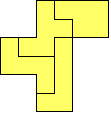# Pentomino Labsby Henri Picciotto

This is an instructional unit. These ideas are developed in much greater detail in my book Working with Pentominoes (Where to get it.) However, to carry them out, you need only plastic pentominoes and one-inch graph paper. Or use my Virtual Pentominoes.

If you're short on time, skip Lab #1. Having students trace and color solutions for Labs #3 and #4 makes for a nice bulletin board exhibit.

## 1. Find the Pentominoes

This introductory activity should precede handing out plastic pentominoes. (See Polyomino Lessons, pp. 3-6.) Students discover the pentominoes on grid paper or Virtual Grid Paper. Younger students could do the activity using interlocking cubes, but to avoid 3D "pentacubes", they need to be warned that all five cubes should touch the table.

An extension is to search for the hexominoes. (Polyomino Lessons, pp. 14-16). Because there are so many of them, you can make this a challenge for the whole class (you keep track of the shapes), or a competition between two teams (a student in each team is in charge of keeping a master list).

## 2. Three-Pentomino Puzzles

The Three-Pentomino Puzzles are a good way to become familiar with the pieces and their names. You keep track of the solutions by listing them on the board using the pentomino names.

An extension is to ask for "two-layer" solutions. (Simultaneous solutions to either puzzle, with no piece used more than once.)

Another introductory activity is to make a two- or even three-layer figure, using two pentominoes for each layer. This is an opportunity to introduce the idea of congruence.

## 3. Pentomino Rectangles

A large-scale project for a class is to search for all possible pentomino rectangles (all sizes).

An extension: find all possible pentomino rectangle pairs. See Simultaneous Pentomino Rectangles for an account of the number of possible solutions (which you don't have to reveal to your students.)

Pentomino rectangles are the subject of pp. 14-23 of Working with Pentominoes.

12-pentomino rectangles are thoroughly discussed in Solomon Golomb's book Polyominoes (Princeton University Press, NJ, 1994.)

## 4. Pentomino Blowups

Another project is to try to cover scaled pentominoes with pentominoes. Print and duplicate the worksheet. Between them, the students should find solutions to as many of the 22 suggested puzzles as possible.

Be sure to discuss questions 2 and 5! This activity alone is not sufficient to establish the relationship between the scaling factor and the ratio of areas, but it helps. For a good introduction to that concept, see Lab 10.3: Polyomino Blowups in my book Geometry Labs.

Extensions:
- Double duplication. Make a figure, using two pentominoes. Make a congruent copy of that figure, using two other pentominoes. Use the remaining eight pentominoes to make a figure that is similar to the first two. There are many solutions to this puzzle, but it is challenging. A more accessible version is to not include the congruent figure.